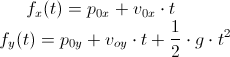# Drawing a projectile trajectory like Angry Birds using LibGDX

We had to implement a projectile trajectory like Angry Birds for our current game and we wanted to share a bit how we did it.

### Introduction

In Angry Birds, the trajectory is drawn after you fired a bird showing its trajectory to help you decide the next shot. Knowing the trajectory of the current projectile wasn't totally needed in that version of the game since you have the slingshot and that tells you, in part, where the current bird is going.

In Angry Birds Space, they changed to show the trajectory of the current bird because they changed the game mechanics and now birds can fly different depending the gravity of the planets, the slingshot doesn't tell you the real direction anymore. So, that was the correct change to help the player with the new rules.

We wanted to test how drawing a trajectory, like Angry Birds Space does for the next shot, could help the player.

### Calculating the trajectory

The first step is to calculate the function f(t) for the projectile trajectory. In our case, projectiles have a normal behavior (there are no mini planets) so the formula is simplified:We found an implementation for the equation in stackoverflow, here the code is:

```class ProjectileEquation {

public float gravity;
public Vector2 startVelocity = new Vector2();
public Vector2 startPoint = new Vector2();

public float getX(float t) {
return startVelocity.x * t + startPoint.x;
}

public float getY(float t) {
return 0.5f * gravity * t * t + startVelocity.y * t + startPoint.y;
}

}
```

With that class we have an easy way to calculate x and y coordinates given the time.

### Drawing it to the screen

If we follow a similar approach of Angry Birds, we can draw colored points for the projectile trajectory.

In our case, we created a LibGDX Actor dedicated to draw the Trajectory of the projectile. It first calculates the trajectory using the previous class and then renders it by using a Sprite and drawing it for each point of the trajectory by using the SpriteBatch's draw method. Here is the code:

```public static class Controller  {

public float power = 50f;
public float angle = 0f;

}

public static class TrajectoryActor extends Actor {

private Controller controller;
private ProjectileEquation projectileEquation;
private Sprite trajectorySprite;

public int trajectoryPointCount = 30;
public float timeSeparation = 1f;

public TrajectoryActor(Controller controller, float gravity, Sprite trajectorySprite) {
this.controller = controller;
this.trajectorySprite = trajectorySprite;
this.projectileEquation = new ProjectileEquation();
this.projectileEquation.gravity = gravity;
}

@Override
public void act(float delta) {
super.act(delta);
projectileEquation.startVelocity.set(controller.power, 0f);
projectileEquation.startVelocity.rotate(controller.angle);
}

@Override
public void draw(SpriteBatch batch, float parentAlpha) {
float t = 0f;
float width = this.width;
float height = this.height;

float timeSeparation = this.timeSeparation;

for (int i = 0; i < trajectoryPointCount; i++) {
float x = this.x + projectileEquation.getX(t);
float y = this.y + projectileEquation.getY(t);

batch.setColor(this.color);
batch.draw(trajectorySprite, x, y, width, height);

t += timeSeparation;
}
}

@Override
public Actor hit(float x, float y) {
return null;
}

}
```

The idea of using the Controller class is to be able to modify the values from outside of the actor by using a shared class between different parts of the code.

### Further improvements

To make it look nicer, one possible addition is to decrement the size of the trajectory points and to reduce their opacity.

In order to do that we drawn each point of the trajectory each time with less alpha in the color and smaller by changing the width and height when calling spritebatch.draw().

We also added a fade in transition to show the trajectory instead making it instantly appear and that works great too, but that is in the game.

Another possible improvement, but depends on the game you are making, is to separate the points using a fixed distance. In order to do that, we have to be dependent on x and not t. So we added a method to the ProjectileEquation class that given a fixed distance and all the values of the class it returns the corresponding t in order to maintain the horizontal distance between points, here is the code:

```	public float getTForGivenX(float x) {
return (x - startPoint.x) / (startVelocity.x);
}
```

Now we can change the draw method of the TrajectoryActor to do, before starting to draw the points:

```	float fixedHorizontalDistance = 10f;
timeSeparation = projectileEquation.getTForGivenX(fixedHorizontalDistance);
```

Not sure which one is the best option between using x or t as the main variable, as I said before, I suppose it depends on the game you are making.

Here is a video showing the results:

If you want to see it working you can test the webstart of the prototypes project, or you can go to the code and see the dirty stuff.

### Conclusion

Making a trajectory if you know the correct formula is not hard and it looks nice, it also could be used to help the players maybe as part of the basic gameplay or maybe as a powerup.

Hope you like it.

VN:F [1.9.22_1171]
Rating: 4.3/5 (22 votes cast)
Drawing a projectile trajectory like Angry Birds using LibGDX, 4.3 out of 5 based on 22 ratings